# ISBA 2016: Foundations

Jun. 17, 2016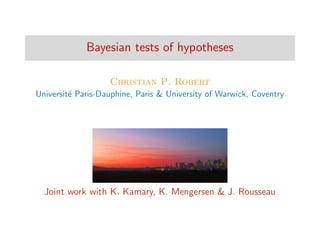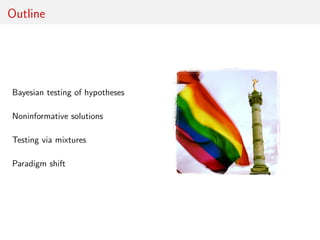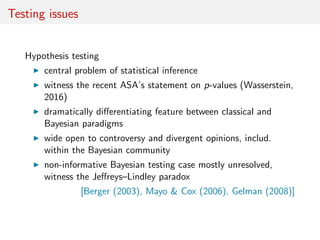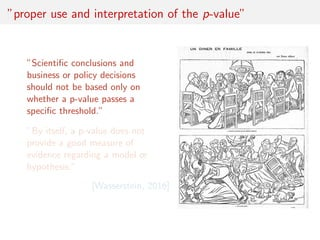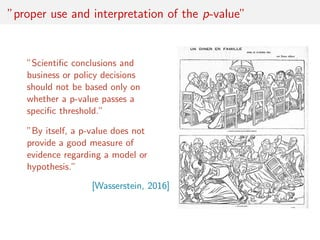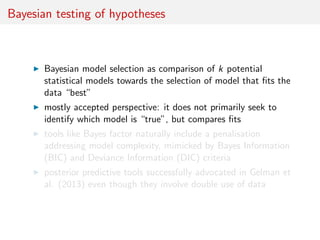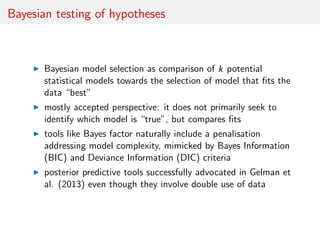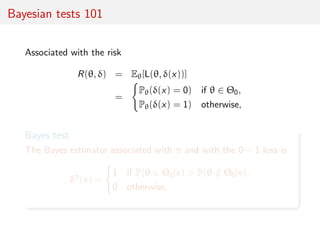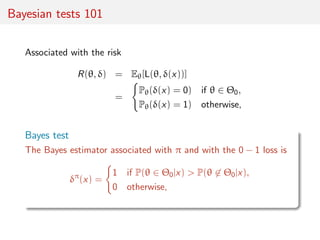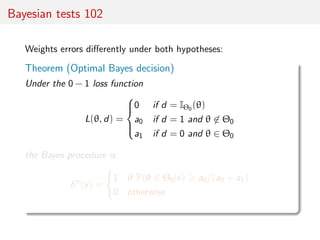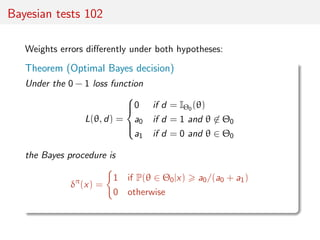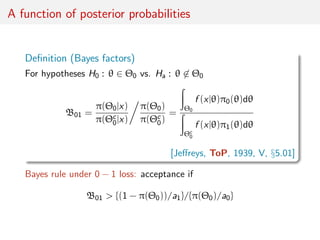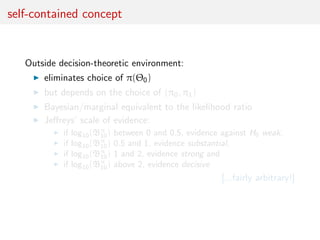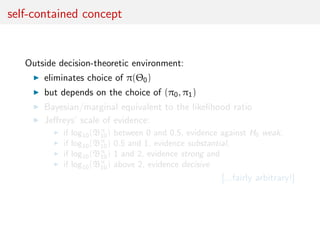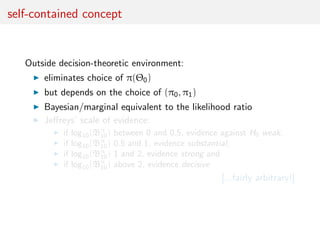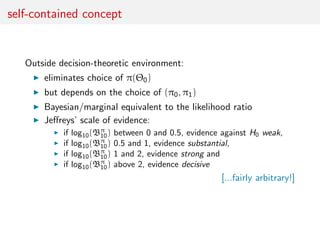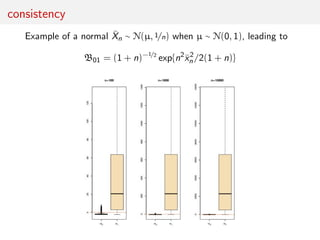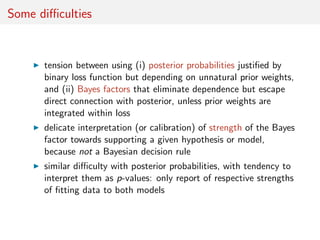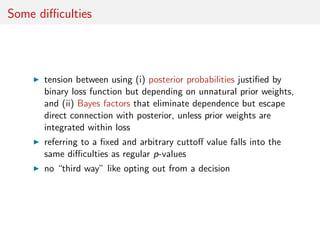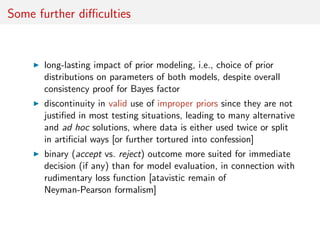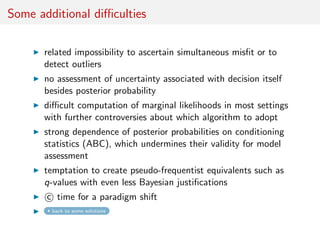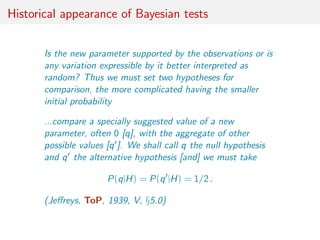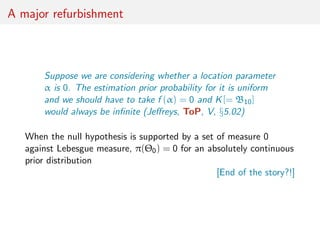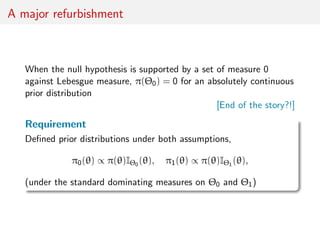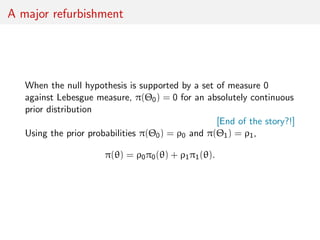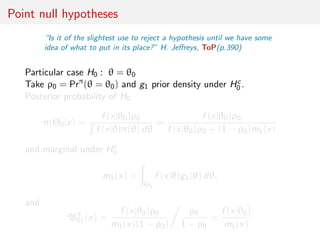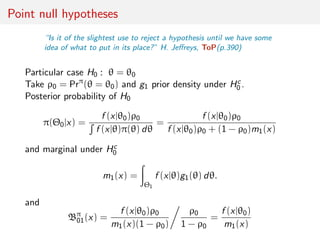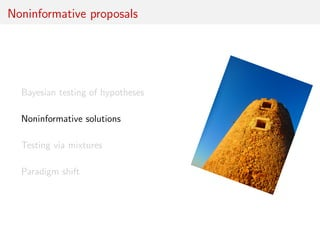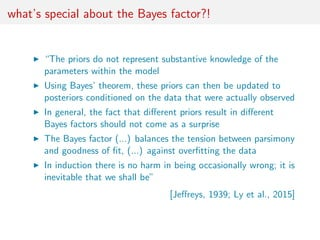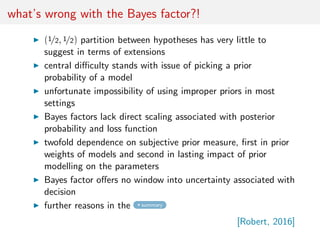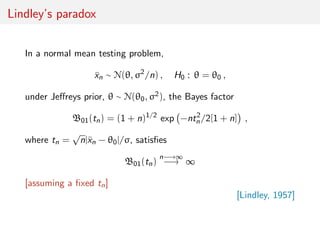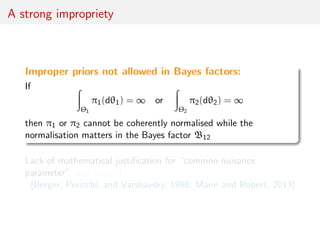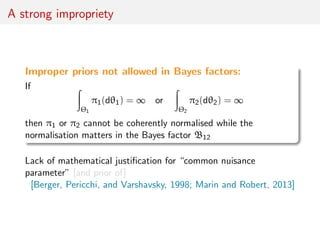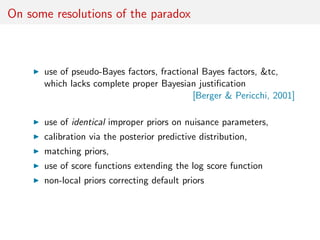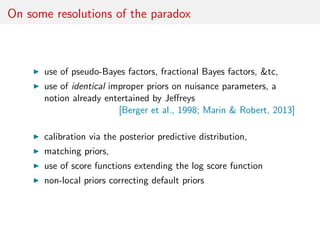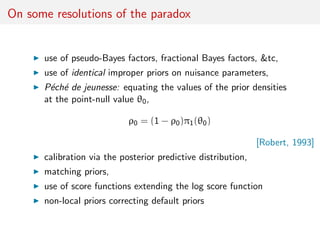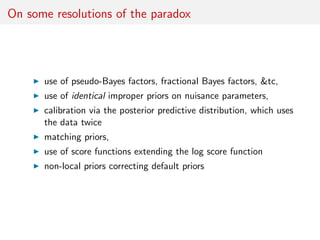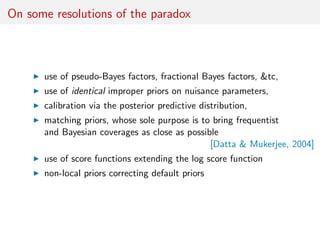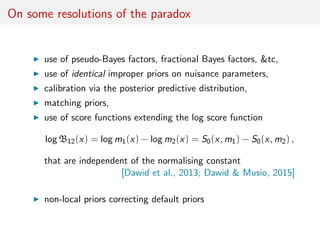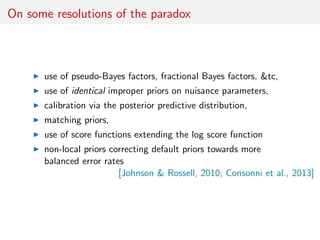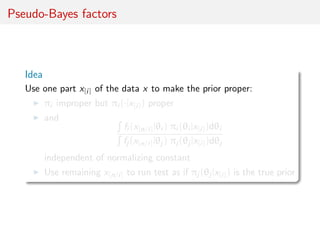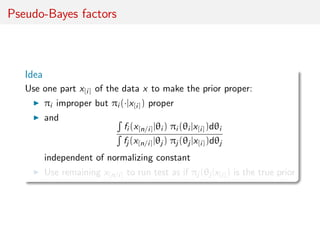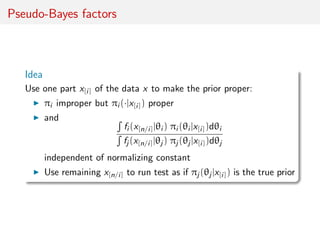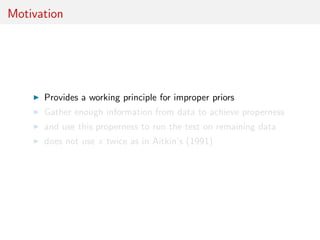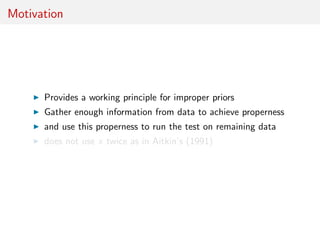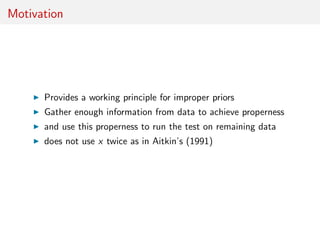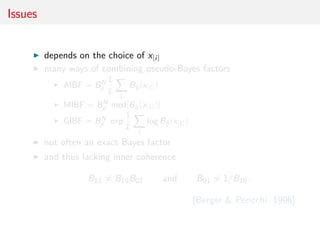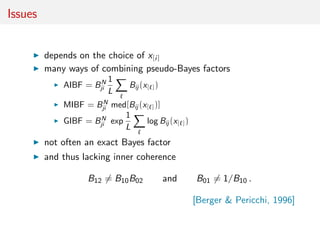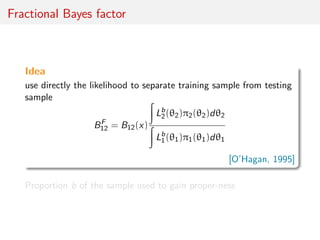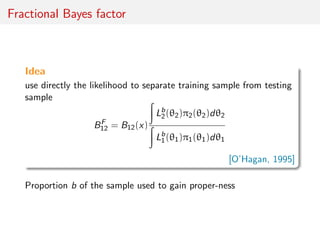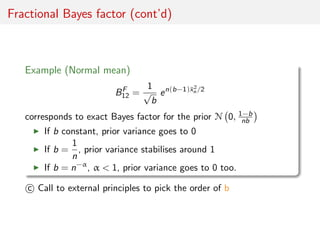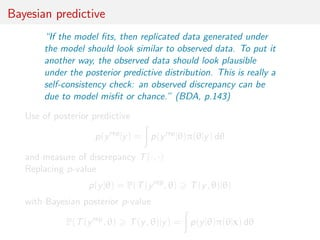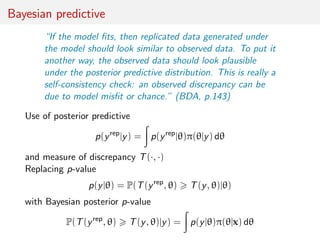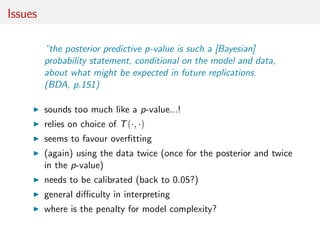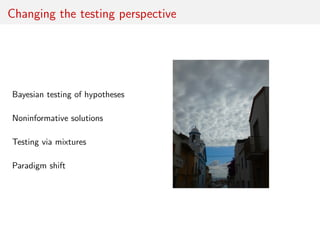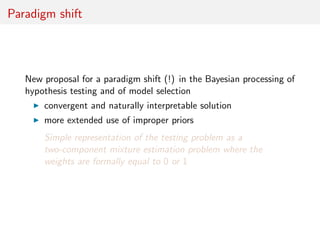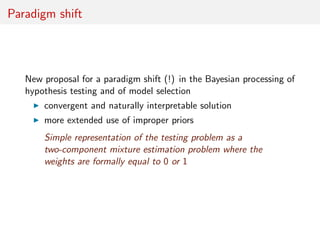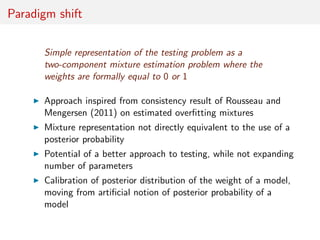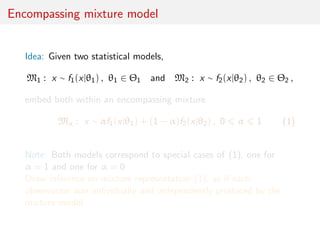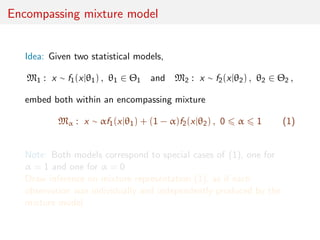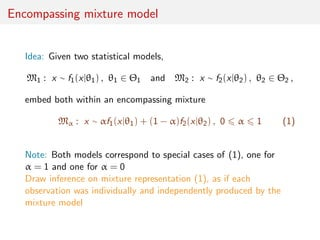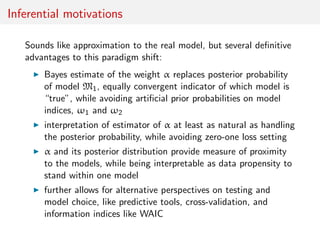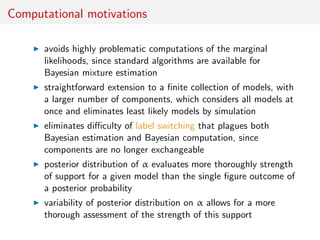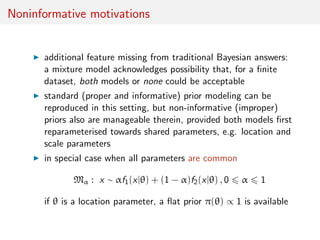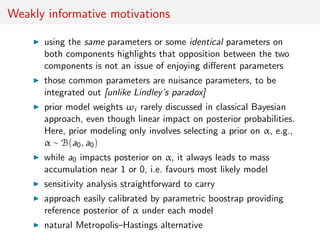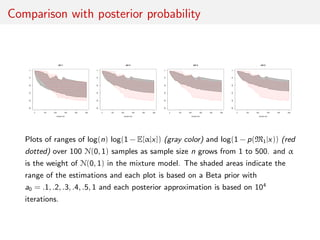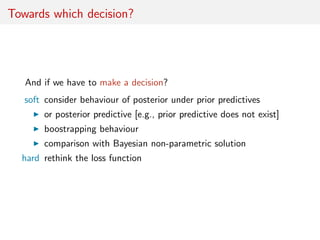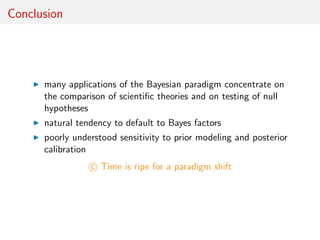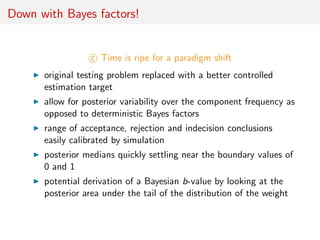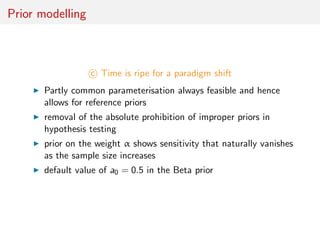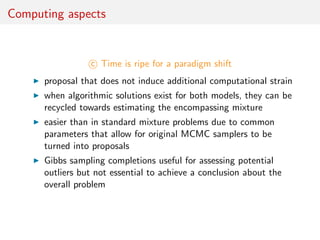1 of 71

### ISBA 2016: Foundations

• 1. Bayesian tests of hypotheses Christian P. Robert Universit´e Paris-Dauphine, Paris & University of Warwick, Coventry Joint work with K. Kamary, K. Mengersen & J. Rousseau
• 2. Outline Bayesian testing of hypotheses Noninformative solutions Testing via mixtures Paradigm shift
• 3. Testing issues Hypothesis testing central problem of statistical inference witness the recent ASA’s statement on p-values (Wasserstein, 2016) dramatically diﬀerentiating feature between classical and Bayesian paradigms wide open to controversy and divergent opinions, includ. within the Bayesian community non-informative Bayesian testing case mostly unresolved, witness the Jeﬀreys–Lindley paradox [Berger (2003), Mayo & Cox (2006), Gelman (2008)]
• 4. ”proper use and interpretation of the p-value” ”Scientiﬁc conclusions and business or policy decisions should not be based only on whether a p-value passes a speciﬁc threshold.” ”By itself, a p-value does not provide a good measure of evidence regarding a model or hypothesis.” [Wasserstein, 2016]
• 5. ”proper use and interpretation of the p-value” ”Scientiﬁc conclusions and business or policy decisions should not be based only on whether a p-value passes a speciﬁc threshold.” ”By itself, a p-value does not provide a good measure of evidence regarding a model or hypothesis.” [Wasserstein, 2016]
• 6. Bayesian testing of hypotheses Bayesian model selection as comparison of k potential statistical models towards the selection of model that ﬁts the data “best” mostly accepted perspective: it does not primarily seek to identify which model is “true”, but compares ﬁts tools like Bayes factor naturally include a penalisation addressing model complexity, mimicked by Bayes Information (BIC) and Deviance Information (DIC) criteria posterior predictive tools successfully advocated in Gelman et al. (2013) even though they involve double use of data
• 7. Bayesian testing of hypotheses Bayesian model selection as comparison of k potential statistical models towards the selection of model that ﬁts the data “best” mostly accepted perspective: it does not primarily seek to identify which model is “true”, but compares ﬁts tools like Bayes factor naturally include a penalisation addressing model complexity, mimicked by Bayes Information (BIC) and Deviance Information (DIC) criteria posterior predictive tools successfully advocated in Gelman et al. (2013) even though they involve double use of data
• 8. Bayesian tests 101 Associated with the risk R(θ, δ) = Eθ[L(θ, δ(x))] = Pθ(δ(x) = 0) if θ ∈ Θ0, Pθ(δ(x) = 1) otherwise, Bayes test The Bayes estimator associated with π and with the 0 − 1 loss is δπ (x) = 1 if P(θ ∈ Θ0|x) > P(θ ∈ Θ0|x), 0 otherwise,
• 9. Bayesian tests 101 Associated with the risk R(θ, δ) = Eθ[L(θ, δ(x))] = Pθ(δ(x) = 0) if θ ∈ Θ0, Pθ(δ(x) = 1) otherwise, Bayes test The Bayes estimator associated with π and with the 0 − 1 loss is δπ (x) = 1 if P(θ ∈ Θ0|x) > P(θ ∈ Θ0|x), 0 otherwise,
• 10. Bayesian tests 102 Weights errors diﬀerently under both hypotheses: Theorem (Optimal Bayes decision) Under the 0 − 1 loss function L(θ, d) =    0 if d = IΘ0 (θ) a0 if d = 1 and θ ∈ Θ0 a1 if d = 0 and θ ∈ Θ0 the Bayes procedure is δπ (x) = 1 if P(θ ∈ Θ0|x) a0/(a0 + a1) 0 otherwise
• 11. Bayesian tests 102 Weights errors diﬀerently under both hypotheses: Theorem (Optimal Bayes decision) Under the 0 − 1 loss function L(θ, d) =    0 if d = IΘ0 (θ) a0 if d = 1 and θ ∈ Θ0 a1 if d = 0 and θ ∈ Θ0 the Bayes procedure is δπ (x) = 1 if P(θ ∈ Θ0|x) a0/(a0 + a1) 0 otherwise
• 12. A function of posterior probabilities Deﬁnition (Bayes factors) For hypotheses H0 : θ ∈ Θ0 vs. Ha : θ ∈ Θ0 B01 = π(Θ0|x) π(Θc 0|x) π(Θ0) π(Θc 0) = Θ0 f (x|θ)π0(θ)dθ Θc 0 f (x|θ)π1(θ)dθ [Jeﬀreys, ToP, 1939, V, §5.01] Bayes rule under 0 − 1 loss: acceptance if B01 > {(1 − π(Θ0))/a1}/{π(Θ0)/a0}
• 13. self-contained concept Outside decision-theoretic environment: eliminates choice of π(Θ0) but depends on the choice of (π0, π1) Bayesian/marginal equivalent to the likelihood ratio Jeﬀreys’ scale of evidence: if log10(Bπ 10) between 0 and 0.5, evidence against H0 weak, if log10(Bπ 10) 0.5 and 1, evidence substantial, if log10(Bπ 10) 1 and 2, evidence strong and if log10(Bπ 10) above 2, evidence decisive [...fairly arbitrary!]
• 14. self-contained concept Outside decision-theoretic environment: eliminates choice of π(Θ0) but depends on the choice of (π0, π1) Bayesian/marginal equivalent to the likelihood ratio Jeﬀreys’ scale of evidence: if log10(Bπ 10) between 0 and 0.5, evidence against H0 weak, if log10(Bπ 10) 0.5 and 1, evidence substantial, if log10(Bπ 10) 1 and 2, evidence strong and if log10(Bπ 10) above 2, evidence decisive [...fairly arbitrary!]
• 15. self-contained concept Outside decision-theoretic environment: eliminates choice of π(Θ0) but depends on the choice of (π0, π1) Bayesian/marginal equivalent to the likelihood ratio Jeﬀreys’ scale of evidence: if log10(Bπ 10) between 0 and 0.5, evidence against H0 weak, if log10(Bπ 10) 0.5 and 1, evidence substantial, if log10(Bπ 10) 1 and 2, evidence strong and if log10(Bπ 10) above 2, evidence decisive [...fairly arbitrary!]
• 16. self-contained concept Outside decision-theoretic environment: eliminates choice of π(Θ0) but depends on the choice of (π0, π1) Bayesian/marginal equivalent to the likelihood ratio Jeﬀreys’ scale of evidence: if log10(Bπ 10) between 0 and 0.5, evidence against H0 weak, if log10(Bπ 10) 0.5 and 1, evidence substantial, if log10(Bπ 10) 1 and 2, evidence strong and if log10(Bπ 10) above 2, evidence decisive [...fairly arbitrary!]
• 17. consistency Example of a normal ¯Xn ∼ N(µ, 1/n) when µ ∼ N(0, 1), leading to B01 = (1 + n)−1/2 exp{n2 ¯x2 n /2(1 + n)}
• 18. Some diﬃculties tension between using (i) posterior probabilities justiﬁed by binary loss function but depending on unnatural prior weights, and (ii) Bayes factors that eliminate dependence but escape direct connection with posterior, unless prior weights are integrated within loss delicate interpretation (or calibration) of strength of the Bayes factor towards supporting a given hypothesis or model, because not a Bayesian decision rule similar diﬃculty with posterior probabilities, with tendency to interpret them as p-values: only report of respective strengths of ﬁtting data to both models
• 19. Some diﬃculties tension between using (i) posterior probabilities justiﬁed by binary loss function but depending on unnatural prior weights, and (ii) Bayes factors that eliminate dependence but escape direct connection with posterior, unless prior weights are integrated within loss referring to a ﬁxed and arbitrary cuttoﬀ value falls into the same diﬃculties as regular p-values no “third way” like opting out from a decision
• 20. Some further diﬃculties long-lasting impact of prior modeling, i.e., choice of prior distributions on parameters of both models, despite overall consistency proof for Bayes factor discontinuity in valid use of improper priors since they are not justiﬁed in most testing situations, leading to many alternative and ad hoc solutions, where data is either used twice or split in artiﬁcial ways [or further tortured into confession] binary (accept vs. reject) outcome more suited for immediate decision (if any) than for model evaluation, in connection with rudimentary loss function [atavistic remain of Neyman-Pearson formalism]
• 21. Some additional diﬃculties related impossibility to ascertain simultaneous misﬁt or to detect outliers no assessment of uncertainty associated with decision itself besides posterior probability diﬃcult computation of marginal likelihoods in most settings with further controversies about which algorithm to adopt strong dependence of posterior probabilities on conditioning statistics (ABC), which undermines their validity for model assessment temptation to create pseudo-frequentist equivalents such as q-values with even less Bayesian justiﬁcations c time for a paradigm shift back to some solutions
• 22. Historical appearance of Bayesian tests Is the new parameter supported by the observations or is any variation expressible by it better interpreted as random? Thus we must set two hypotheses for comparison, the more complicated having the smaller initial probability ...compare a specially suggested value of a new parameter, often 0 [q], with the aggregate of other possible values [q ]. We shall call q the null hypothesis and q the alternative hypothesis [and] we must take P(q|H) = P(q |H) = 1/2 . (Jeﬀreys, ToP, 1939, V, §5.0)
• 23. A major refurbishment Suppose we are considering whether a location parameter α is 0. The estimation prior probability for it is uniform and we should have to take f (α) = 0 and K[= B10] would always be inﬁnite (Jeﬀreys, ToP, V, §5.02) When the null hypothesis is supported by a set of measure 0 against Lebesgue measure, π(Θ0) = 0 for an absolutely continuous prior distribution [End of the story?!]
• 24. A major refurbishment When the null hypothesis is supported by a set of measure 0 against Lebesgue measure, π(Θ0) = 0 for an absolutely continuous prior distribution [End of the story?!] Requirement Deﬁned prior distributions under both assumptions, π0(θ) ∝ π(θ)IΘ0 (θ), π1(θ) ∝ π(θ)IΘ1 (θ), (under the standard dominating measures on Θ0 and Θ1)
• 25. A major refurbishment When the null hypothesis is supported by a set of measure 0 against Lebesgue measure, π(Θ0) = 0 for an absolutely continuous prior distribution [End of the story?!] Using the prior probabilities π(Θ0) = ρ0 and π(Θ1) = ρ1, π(θ) = ρ0π0(θ) + ρ1π1(θ).
• 26. Point null hypotheses “Is it of the slightest use to reject a hypothesis until we have some idea of what to put in its place?” H. Jeﬀreys, ToP(p.390) Particular case H0 : θ = θ0 Take ρ0 = Prπ (θ = θ0) and g1 prior density under Hc 0 . Posterior probability of H0 π(Θ0|x) = f (x|θ0)ρ0 f (x|θ)π(θ) dθ = f (x|θ0)ρ0 f (x|θ0)ρ0 + (1 − ρ0)m1(x) and marginal under Hc 0 m1(x) = Θ1 f (x|θ)g1(θ) dθ. and Bπ 01(x) = f (x|θ0)ρ0 m1(x)(1 − ρ0) ρ0 1 − ρ0 = f (x|θ0) m1(x)
• 27. Point null hypotheses “Is it of the slightest use to reject a hypothesis until we have some idea of what to put in its place?” H. Jeﬀreys, ToP(p.390) Particular case H0 : θ = θ0 Take ρ0 = Prπ (θ = θ0) and g1 prior density under Hc 0 . Posterior probability of H0 π(Θ0|x) = f (x|θ0)ρ0 f (x|θ)π(θ) dθ = f (x|θ0)ρ0 f (x|θ0)ρ0 + (1 − ρ0)m1(x) and marginal under Hc 0 m1(x) = Θ1 f (x|θ)g1(θ) dθ. and Bπ 01(x) = f (x|θ0)ρ0 m1(x)(1 − ρ0) ρ0 1 − ρ0 = f (x|θ0) m1(x)
• 28. Noninformative proposals Bayesian testing of hypotheses Noninformative solutions Testing via mixtures Paradigm shift
• 29. what’s special about the Bayes factor?! “The priors do not represent substantive knowledge of the parameters within the model Using Bayes’ theorem, these priors can then be updated to posteriors conditioned on the data that were actually observed In general, the fact that diﬀerent priors result in diﬀerent Bayes factors should not come as a surprise The Bayes factor (...) balances the tension between parsimony and goodness of ﬁt, (...) against overﬁtting the data In induction there is no harm in being occasionally wrong; it is inevitable that we shall be” [Jeﬀreys, 1939; Ly et al., 2015]
• 30. what’s wrong with the Bayes factor?! (1/2, 1/2) partition between hypotheses has very little to suggest in terms of extensions central diﬃculty stands with issue of picking a prior probability of a model unfortunate impossibility of using improper priors in most settings Bayes factors lack direct scaling associated with posterior probability and loss function twofold dependence on subjective prior measure, ﬁrst in prior weights of models and second in lasting impact of prior modelling on the parameters Bayes factor oﬀers no window into uncertainty associated with decision further reasons in the summary [Robert, 2016]
• 31. Lindley’s paradox In a normal mean testing problem, ¯xn ∼ N(θ, σ2 /n) , H0 : θ = θ0 , under Jeﬀreys prior, θ ∼ N(θ0, σ2), the Bayes factor B01(tn) = (1 + n)1/2 exp −nt2 n/2[1 + n] , where tn = √ n|¯xn − θ0|/σ, satisﬁes B01(tn) n−→∞ −→ ∞ [assuming a ﬁxed tn] [Lindley, 1957]
• 32. A strong impropriety Improper priors not allowed in Bayes factors: If Θ1 π1(dθ1) = ∞ or Θ2 π2(dθ2) = ∞ then π1 or π2 cannot be coherently normalised while the normalisation matters in the Bayes factor B12 Lack of mathematical justiﬁcation for “common nuisance parameter” [and prior of] [Berger, Pericchi, and Varshavsky, 1998; Marin and Robert, 2013]
• 33. A strong impropriety Improper priors not allowed in Bayes factors: If Θ1 π1(dθ1) = ∞ or Θ2 π2(dθ2) = ∞ then π1 or π2 cannot be coherently normalised while the normalisation matters in the Bayes factor B12 Lack of mathematical justiﬁcation for “common nuisance parameter” [and prior of] [Berger, Pericchi, and Varshavsky, 1998; Marin and Robert, 2013]
• 34. On some resolutions of the paradox use of pseudo-Bayes factors, fractional Bayes factors, &tc, which lacks complete proper Bayesian justiﬁcation [Berger & Pericchi, 2001] use of identical improper priors on nuisance parameters, calibration via the posterior predictive distribution, matching priors, use of score functions extending the log score function non-local priors correcting default priors
• 35. On some resolutions of the paradox use of pseudo-Bayes factors, fractional Bayes factors, &tc, use of identical improper priors on nuisance parameters, a notion already entertained by Jeﬀreys [Berger et al., 1998; Marin & Robert, 2013] calibration via the posterior predictive distribution, matching priors, use of score functions extending the log score function non-local priors correcting default priors
• 36. On some resolutions of the paradox use of pseudo-Bayes factors, fractional Bayes factors, &tc, use of identical improper priors on nuisance parameters, P´ech´e de jeunesse: equating the values of the prior densities at the point-null value θ0, ρ0 = (1 − ρ0)π1(θ0) [Robert, 1993] calibration via the posterior predictive distribution, matching priors, use of score functions extending the log score function non-local priors correcting default priors
• 37. On some resolutions of the paradox use of pseudo-Bayes factors, fractional Bayes factors, &tc, use of identical improper priors on nuisance parameters, calibration via the posterior predictive distribution, which uses the data twice matching priors, use of score functions extending the log score function non-local priors correcting default priors
• 38. On some resolutions of the paradox use of pseudo-Bayes factors, fractional Bayes factors, &tc, use of identical improper priors on nuisance parameters, calibration via the posterior predictive distribution, matching priors, whose sole purpose is to bring frequentist and Bayesian coverages as close as possible [Datta & Mukerjee, 2004] use of score functions extending the log score function non-local priors correcting default priors
• 39. On some resolutions of the paradox use of pseudo-Bayes factors, fractional Bayes factors, &tc, use of identical improper priors on nuisance parameters, calibration via the posterior predictive distribution, matching priors, use of score functions extending the log score function log B12(x) = log m1(x) − log m2(x) = S0(x, m1) − S0(x, m2) , that are independent of the normalising constant [Dawid et al., 2013; Dawid & Musio, 2015] non-local priors correcting default priors
• 40. On some resolutions of the paradox use of pseudo-Bayes factors, fractional Bayes factors, &tc, use of identical improper priors on nuisance parameters, calibration via the posterior predictive distribution, matching priors, use of score functions extending the log score function non-local priors correcting default priors towards more balanced error rates [Johnson & Rossell, 2010; Consonni et al., 2013]
• 41. Pseudo-Bayes factors Idea Use one part x[i] of the data x to make the prior proper: πi improper but πi (·|x[i]) proper and fi (x[n/i]|θi ) πi (θi |x[i])dθi fj (x[n/i]|θj ) πj (θj |x[i])dθj independent of normalizing constant Use remaining x[n/i] to run test as if πj (θj |x[i]) is the true prior
• 42. Pseudo-Bayes factors Idea Use one part x[i] of the data x to make the prior proper: πi improper but πi (·|x[i]) proper and fi (x[n/i]|θi ) πi (θi |x[i])dθi fj (x[n/i]|θj ) πj (θj |x[i])dθj independent of normalizing constant Use remaining x[n/i] to run test as if πj (θj |x[i]) is the true prior
• 43. Pseudo-Bayes factors Idea Use one part x[i] of the data x to make the prior proper: πi improper but πi (·|x[i]) proper and fi (x[n/i]|θi ) πi (θi |x[i])dθi fj (x[n/i]|θj ) πj (θj |x[i])dθj independent of normalizing constant Use remaining x[n/i] to run test as if πj (θj |x[i]) is the true prior
• 44. Motivation Provides a working principle for improper priors Gather enough information from data to achieve properness and use this properness to run the test on remaining data does not use x twice as in Aitkin’s (1991)
• 45. Motivation Provides a working principle for improper priors Gather enough information from data to achieve properness and use this properness to run the test on remaining data does not use x twice as in Aitkin’s (1991)
• 46. Motivation Provides a working principle for improper priors Gather enough information from data to achieve properness and use this properness to run the test on remaining data does not use x twice as in Aitkin’s (1991)
• 47. Issues depends on the choice of x[i] many ways of combining pseudo-Bayes factors AIBF = BN ji 1 L Bij (x[ ]) MIBF = BN ji med[Bij (x[ ])] GIBF = BN ji exp 1 L log Bij (x[ ]) not often an exact Bayes factor and thus lacking inner coherence B12 = B10B02 and B01 = 1/B10 . [Berger & Pericchi, 1996]
• 48. Issues depends on the choice of x[i] many ways of combining pseudo-Bayes factors AIBF = BN ji 1 L Bij (x[ ]) MIBF = BN ji med[Bij (x[ ])] GIBF = BN ji exp 1 L log Bij (x[ ]) not often an exact Bayes factor and thus lacking inner coherence B12 = B10B02 and B01 = 1/B10 . [Berger & Pericchi, 1996]
• 49. Fractional Bayes factor Idea use directly the likelihood to separate training sample from testing sample BF 12 = B12(x) Lb 2(θ2)π2(θ2)dθ2 Lb 1(θ1)π1(θ1)dθ1 [O’Hagan, 1995] Proportion b of the sample used to gain proper-ness
• 50. Fractional Bayes factor Idea use directly the likelihood to separate training sample from testing sample BF 12 = B12(x) Lb 2(θ2)π2(θ2)dθ2 Lb 1(θ1)π1(θ1)dθ1 [O’Hagan, 1995] Proportion b of the sample used to gain proper-ness
• 51. Fractional Bayes factor (cont’d) Example (Normal mean) BF 12 = 1 √ b en(b−1)¯x2 n /2 corresponds to exact Bayes factor for the prior N 0, 1−b nb If b constant, prior variance goes to 0 If b = 1 n , prior variance stabilises around 1 If b = n−α , α < 1, prior variance goes to 0 too. c Call to external principles to pick the order of b
• 52. Bayesian predictive “If the model ﬁts, then replicated data generated under the model should look similar to observed data. To put it another way, the observed data should look plausible under the posterior predictive distribution. This is really a self-consistency check: an observed discrepancy can be due to model misﬁt or chance.” (BDA, p.143) Use of posterior predictive p(yrep |y) = p(yrep |θ)π(θ|y) dθ and measure of discrepancy T(·, ·) Replacing p-value p(y|θ) = P(T(yrep , θ) T(y, θ)|θ) with Bayesian posterior p-value P(T(yrep , θ) T(y, θ)|y) = p(y|θ)π(θ|x) dθ
• 53. Bayesian predictive “If the model ﬁts, then replicated data generated under the model should look similar to observed data. To put it another way, the observed data should look plausible under the posterior predictive distribution. This is really a self-consistency check: an observed discrepancy can be due to model misﬁt or chance.” (BDA, p.143) Use of posterior predictive p(yrep |y) = p(yrep |θ)π(θ|y) dθ and measure of discrepancy T(·, ·) Replacing p-value p(y|θ) = P(T(yrep , θ) T(y, θ)|θ) with Bayesian posterior p-value P(T(yrep , θ) T(y, θ)|y) = p(y|θ)π(θ|x) dθ
• 54. Issues “the posterior predictive p-value is such a [Bayesian] probability statement, conditional on the model and data, about what might be expected in future replications. (BDA, p.151) sounds too much like a p-value...! relies on choice of T(·, ·) seems to favour overﬁtting (again) using the data twice (once for the posterior and twice in the p-value) needs to be calibrated (back to 0.05?) general diﬃculty in interpreting where is the penalty for model complexity?
• 55. Changing the testing perspective Bayesian testing of hypotheses Noninformative solutions Testing via mixtures Paradigm shift
• 56. Paradigm shift New proposal for a paradigm shift (!) in the Bayesian processing of hypothesis testing and of model selection convergent and naturally interpretable solution more extended use of improper priors Simple representation of the testing problem as a two-component mixture estimation problem where the weights are formally equal to 0 or 1
• 57. Paradigm shift New proposal for a paradigm shift (!) in the Bayesian processing of hypothesis testing and of model selection convergent and naturally interpretable solution more extended use of improper priors Simple representation of the testing problem as a two-component mixture estimation problem where the weights are formally equal to 0 or 1
• 58. Paradigm shift Simple representation of the testing problem as a two-component mixture estimation problem where the weights are formally equal to 0 or 1 Approach inspired from consistency result of Rousseau and Mengersen (2011) on estimated overﬁtting mixtures Mixture representation not directly equivalent to the use of a posterior probability Potential of a better approach to testing, while not expanding number of parameters Calibration of posterior distribution of the weight of a model, moving from artiﬁcial notion of posterior probability of a model
• 59. Encompassing mixture model Idea: Given two statistical models, M1 : x ∼ f1(x|θ1) , θ1 ∈ Θ1 and M2 : x ∼ f2(x|θ2) , θ2 ∈ Θ2 , embed both within an encompassing mixture Mα : x ∼ αf1(x|θ1) + (1 − α)f2(x|θ2) , 0 α 1 (1) Note: Both models correspond to special cases of (1), one for α = 1 and one for α = 0 Draw inference on mixture representation (1), as if each observation was individually and independently produced by the mixture model
• 60. Encompassing mixture model Idea: Given two statistical models, M1 : x ∼ f1(x|θ1) , θ1 ∈ Θ1 and M2 : x ∼ f2(x|θ2) , θ2 ∈ Θ2 , embed both within an encompassing mixture Mα : x ∼ αf1(x|θ1) + (1 − α)f2(x|θ2) , 0 α 1 (1) Note: Both models correspond to special cases of (1), one for α = 1 and one for α = 0 Draw inference on mixture representation (1), as if each observation was individually and independently produced by the mixture model
• 61. Encompassing mixture model Idea: Given two statistical models, M1 : x ∼ f1(x|θ1) , θ1 ∈ Θ1 and M2 : x ∼ f2(x|θ2) , θ2 ∈ Θ2 , embed both within an encompassing mixture Mα : x ∼ αf1(x|θ1) + (1 − α)f2(x|θ2) , 0 α 1 (1) Note: Both models correspond to special cases of (1), one for α = 1 and one for α = 0 Draw inference on mixture representation (1), as if each observation was individually and independently produced by the mixture model
• 62. Inferential motivations Sounds like approximation to the real model, but several deﬁnitive advantages to this paradigm shift: Bayes estimate of the weight α replaces posterior probability of model M1, equally convergent indicator of which model is “true”, while avoiding artiﬁcial prior probabilities on model indices, ω1 and ω2 interpretation of estimator of α at least as natural as handling the posterior probability, while avoiding zero-one loss setting α and its posterior distribution provide measure of proximity to the models, while being interpretable as data propensity to stand within one model further allows for alternative perspectives on testing and model choice, like predictive tools, cross-validation, and information indices like WAIC
• 63. Computational motivations avoids highly problematic computations of the marginal likelihoods, since standard algorithms are available for Bayesian mixture estimation straightforward extension to a ﬁnite collection of models, with a larger number of components, which considers all models at once and eliminates least likely models by simulation eliminates diﬃculty of label switching that plagues both Bayesian estimation and Bayesian computation, since components are no longer exchangeable posterior distribution of α evaluates more thoroughly strength of support for a given model than the single ﬁgure outcome of a posterior probability variability of posterior distribution on α allows for a more thorough assessment of the strength of this support
• 64. Noninformative motivations additional feature missing from traditional Bayesian answers: a mixture model acknowledges possibility that, for a ﬁnite dataset, both models or none could be acceptable standard (proper and informative) prior modeling can be reproduced in this setting, but non-informative (improper) priors also are manageable therein, provided both models ﬁrst reparameterised towards shared parameters, e.g. location and scale parameters in special case when all parameters are common Mα : x ∼ αf1(x|θ) + (1 − α)f2(x|θ) , 0 α 1 if θ is a location parameter, a ﬂat prior π(θ) ∝ 1 is available
• 65. Weakly informative motivations using the same parameters or some identical parameters on both components highlights that opposition between the two components is not an issue of enjoying diﬀerent parameters those common parameters are nuisance parameters, to be integrated out [unlike Lindley’s paradox] prior model weights ωi rarely discussed in classical Bayesian approach, even though linear impact on posterior probabilities. Here, prior modeling only involves selecting a prior on α, e.g., α ∼ B(a0, a0) while a0 impacts posterior on α, it always leads to mass accumulation near 1 or 0, i.e. favours most likely model sensitivity analysis straightforward to carry approach easily calibrated by parametric boostrap providing reference posterior of α under each model natural Metropolis–Hastings alternative
• 66. Comparison with posterior probability 0 100 200 300 400 500 -50-40-30-20-100 a0=.1 sample size 0 100 200 300 400 500 -50-40-30-20-100 a0=.3 sample size 0 100 200 300 400 500 -50-40-30-20-100 a0=.4 sample size 0 100 200 300 400 500 -50-40-30-20-100 a0=.5 sample size Plots of ranges of log(n) log(1 − E[α|x]) (gray color) and log(1 − p(M1|x)) (red dotted) over 100 N(0, 1) samples as sample size n grows from 1 to 500. and α is the weight of N(0, 1) in the mixture model. The shaded areas indicate the range of the estimations and each plot is based on a Beta prior with a0 = .1, .2, .3, .4, .5, 1 and each posterior approximation is based on 104 iterations.
• 67. Towards which decision? And if we have to make a decision? soft consider behaviour of posterior under prior predictives or posterior predictive [e.g., prior predictive does not exist] boostrapping behaviour comparison with Bayesian non-parametric solution hard rethink the loss function
• 68. Conclusion many applications of the Bayesian paradigm concentrate on the comparison of scientiﬁc theories and on testing of null hypotheses natural tendency to default to Bayes factors poorly understood sensitivity to prior modeling and posterior calibration c Time is ripe for a paradigm shift
• 69. Down with Bayes factors! c Time is ripe for a paradigm shift original testing problem replaced with a better controlled estimation target allow for posterior variability over the component frequency as opposed to deterministic Bayes factors range of acceptance, rejection and indecision conclusions easily calibrated by simulation posterior medians quickly settling near the boundary values of 0 and 1 potential derivation of a Bayesian b-value by looking at the posterior area under the tail of the distribution of the weight
• 70. Prior modelling c Time is ripe for a paradigm shift Partly common parameterisation always feasible and hence allows for reference priors removal of the absolute prohibition of improper priors in hypothesis testing prior on the weight α shows sensitivity that naturally vanishes as the sample size increases default value of a0 = 0.5 in the Beta prior
• 71. Computing aspects c Time is ripe for a paradigm shift proposal that does not induce additional computational strain when algorithmic solutions exist for both models, they can be recycled towards estimating the encompassing mixture easier than in standard mixture problems due to common parameters that allow for original MCMC samplers to be turned into proposals Gibbs sampling completions useful for assessing potential outliers but not essential to achieve a conclusion about the overall problem